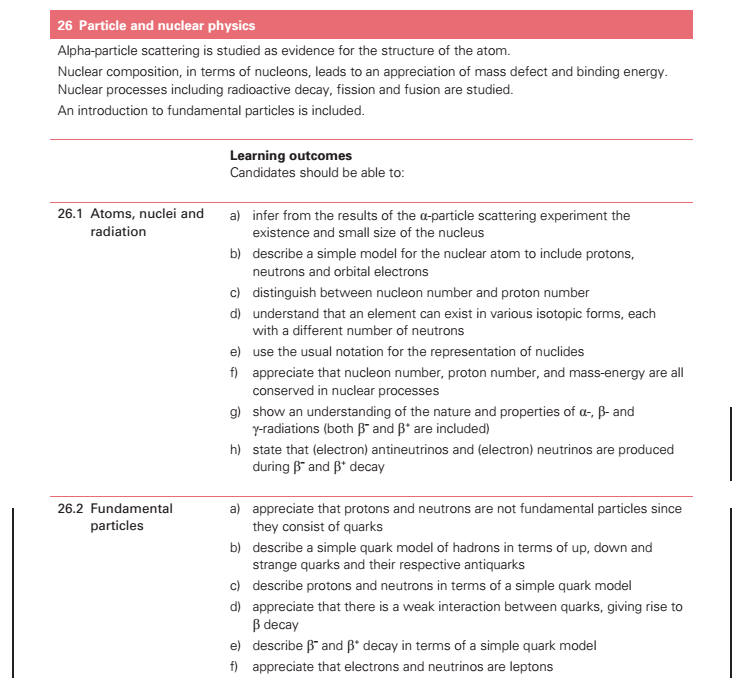26.1 Atoms, nuclei and radiation   a) infer from the results of the alpha scattering[] experiment the existence and small size of the nucleus b) describe a simple model for the nuclear atom to include protons,  neutrons and orbital electrons c) distinguish between nucleon number and proton number d) understand that an element can exist in various isotopic forms, each  with a different number of neutrons e) use the usual notation for the representation of nuclides f) appreciate that nucleon number, proton number, and mass-energy are all  conserved in nuclear processes g) show an understanding of the nature and properties of α-, β- and  γ-radiations (both β – and β + are included) h) state that (electron) antineutrinos and (electron) neutrinos are produced  during β – and β + decay 26.2 Fundamental  particles a) appreciate that protons and neutrons are not fundamental particles since  they consist of quarks b) describe a simple quark model of hadrons in terms of up, down and  strange quarks and their respective antiquarks c) describe protons and neutrons in terms of a simple quark model d) appreciate that there is a weak interaction between quarks, giving rise to beta decay e) describe β – and β + decay in terms of a simple quark model f) appreciate that electrons and neutrinos are leptons